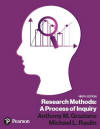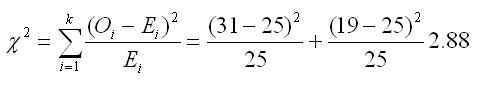﻿ Manual Computation of the Chi Square Goodness-of-Fit TestGraziano & Raulin
Research Methods (9th edition)

## Chi Square Goodness of Fit Test

Problem: Suppose that in an ESP experiment the participant is to guess whether the experimenter is looking at a picture of a car or a picture of a tree. Each picture appears 25 times in random order. The participant guesses correctly 31 times and incorrectly 19 times. Do these data suggest that the person may have ESP?

Because the participant had a 50/50 chance of guessing correctly, we expect 25 correct guesses and 25 incorrect guesses by chance, with variations from that even split the result of sampling bias. Therefore, we set up the data matrix like so:

 Correct Incorrect Observed 31 19 Expected 25 25

We compute the value of chi-square using the formula shown below.The calculated value of chi-square is compared with a critical value from the Chi-Square Table. With an alpha of 0.05, degrees of freedom equal to the number of categories (in this case 2) minus 1, the critical value is 3.841.

Because the observed chi-square (2.88) is less than the critical value (3.841), we fail to reject the null hypothesis and conclude there is not sufficient evidence to suggest the person tested has ESP powers.